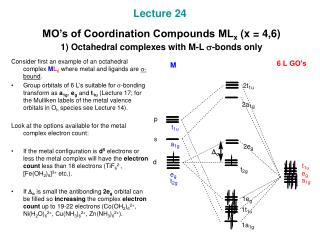DownloadDownload PresentationLecture 24 MO’s of Coordination Compounds ML x (x = 4,6) 1) Octahedral complexes with M-L s -bonds only

# Lecture 24 MO’s of Coordination Compounds ML x (x = 4,6) 1) Octahedral complexes with M-L s -bonds only

Télécharger la présentation## Lecture 24 MO’s of Coordination Compounds ML x (x = 4,6) 1) Octahedral complexes with M-L s -bonds only

- - - - - - - - - - - - - - - - - - - - - - - - - - - E N D - - - - - - - - - - - - - - - - - - - - - - - - - - -
##### Presentation Transcript

1. Lecture 24 MO’s of Coordination Compounds MLx (x = 4,6) 1) Octahedral complexes with M-L s-bonds only Consider first an example of an octahedral complex ML6 where metal and ligands are s-bound. • Group orbitals of 6 L’s suitable for s-bonding transform as a1g, eg and t1u (Lecture 17; for the Mulliken labels of the metal valence orbitals in Oh species see Lecture 14). Look at the options available for the metal complex electron count: • If the metal configuration is d5 electrons or less the metal complex will have the electron count less than 18 electrons (TiF62-, [Fe(OH2)6]3+ etc.). • If Do is small the antibonding 2eg orbital can be filled so increasing the complex electron count up to 19-22 electrons (Co(OH2)62+, Ni(H2O)62+, Cu(NH3)62+, Zn(NH3)62+).

2. 2) Octahedral complexes with M-L p-bonds. p-Donating ligands The symmetry of metal and ligand group orbitals suitable for M-Lp-bonding in octahedral complexes can be found using group theory: Gr(p) = T1g + T2g + T1u + T2u (see Lecture 17). • Transition metal atom has orbitals of the t2g(dxy, dyz, dxz) and t1u (px, py, pz) symmetry and no orbitals of the t1g or t2u (see the character table below). • Metal orbitals of the t1u symmetry are already involved in s-bonding with 6 L’s (see the previous diagram). • Therefore, p-bonding is only possible between metal and ligand orbitals of the t2g symmetry. Consider the case of the ligand-to-metal p-donation when the ligands t2g orbitals are fully filled (and of low energy) while the metal t2g orbitals are not completely filled. • We will get two new t2g-MO’s, 1t2g and 2t2g. • The energy gap between the partially filled 2t2g and eg MO’s and thus Do are now smaller. • So p-donating ligand becomes a weak field ligand (halogeno ligands, OH-, H2O etc).

3. 3) Octahedral complexes with M-L p-bonds. p-Accepting ligands • In the case of p-accepting ligands like CO, CN- etc. the ligands group orbitals of t2g symmetry are empty and higher in energy than corresponding t2g metal orbitals. • The metal-ligand p-bonding stabilizes the metal complex and increases Do. • Therefore the ability of a ligand to be a p-acceptor makes the ligand a stronger field ligand. • Increased Do prevents the eg level to be filled and the metal valence shell to be “overfilled” and helps it obey 18 electron rule.

4. 4) Tetrahedral complexes with M-L s- and p-bonds Consider first the case of s-only bonded tetrahedral metal complex ML4. • The ligand group orbitals of a1 and t2 symmetry are similar to those considered for 4H GO’s (Lecture 17); the Mulliken labels for the metal valence orbitals in a Td species were assigned (Lecture 14). • The 2tg MO is antibonding and that is why the electron count less than 18 is quite common for ML4 (FeCl4-, CoCl42- etc.). • From the other hand, Dt is usually small and the electron count up to 18 electrons for ML4 is possible but rare. The case of tetrahedral metal complex ML4 with M-Lp-bonds. • The orbitals of e (dz2, dx2-y2), t1 and t2 (px, py, pz; dxz, dyz, dxy) symmetry are suitable for p-bonding with L’s (Lecture 14). • Two e metal orbitals are only available to form M-L p-bonds since the t2 orbitals are involved in M-L s-bonding. • p-acceptors will stabilize MO’s of e-symmetry so increasing Dt (not shown on the diagram).